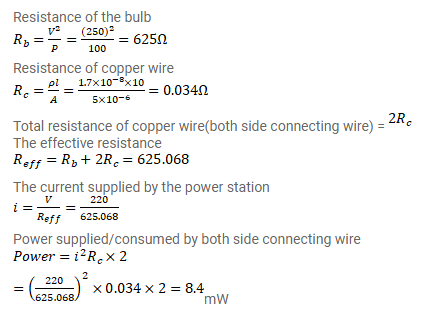# A bulb with rating 250V,

Question:

A bulb with rating $250 \mathrm{~V}, 100 \mathrm{~W}$ is connected to a power supply of $220 \mathrm{~V}$ situated $10 \mathrm{~m}$ away using a copper wire of area pf cross-section $5 \mathrm{~mm}^{2}$. How much power will be consumed by the connecting wires? Resistivity of copper $=1.7 \times 10^{-8} \Omega-m$.

Solution: